# Add formulas OUTSIDE Pivot Tables = Inefficiency

Why do we add formulas outside Pivot Tables?  Because we think Pivot Table cannot do the calculation. Did you ever check if it can?
Or did you just ASSUME?

Here is your chance to find out!## Why do we put formulas outside Pivot Tables?

Because we want to get some output which you think Pivot Table itself cannot give you. This assumption is usually baseless because we have not even tried to find out if Pivot Table can do it.

Once you explore what Pivot Table CAN do, you will be amazed. There are many things in Pivot Table. But we are going to discuss four specific things.

1. Show Values As
2. Grouping
3. Calculated Fields
4. Calculated Items

In this article we will see some options from Show Values As.

## Problems with manually added formulas

Shown in the image above, we want percentage breakup in the third column. Therefore we added a manual formula. The manually added formula goes outdated if you filter something. Now the grand total moved one row up – so all your formulas are showing #DIV/0 error.

Another problem is that if the pivot table expands, it will overwrite your formulas.

Finally, if Pivot table becomes complex, your formulas will also need to be made more complex – which is extra manual work.

In short, unless it is proven that Pivot Table cannot do what you want, DO NOT use formulas outside pivot tables.

Let us explore Show Value As feature.

## Show values as

This feature was earlier in Value Field Settings – Show values As tab for more than a decade.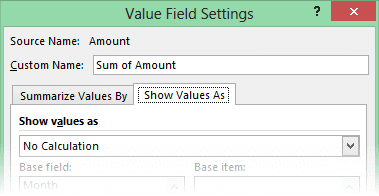Nobody noticed it. So Microsoft added it to the right click menu in data area since 2010. A few people did notice it – But almost nobody noticed ALL options. So let us notice them.

There is Summarize By and Show Values As.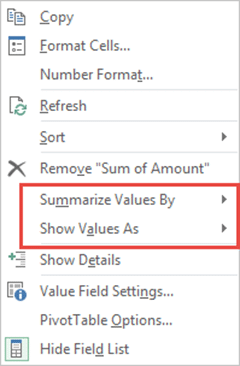Summarization is done automatically. If you drag drop an numeric column in data area the default summarization is SUM. You can change it to other options like Average, Min, Max, etc.

Sometimes, even if you drag-drop a numeric column in data area, it calculates COUNT. This basically indicates that at least ONE value in the column is NOT A NUMBER!

But we are interested in Show Values As.
Excel is asking you – “how do you wan to show the values with respect to each other?”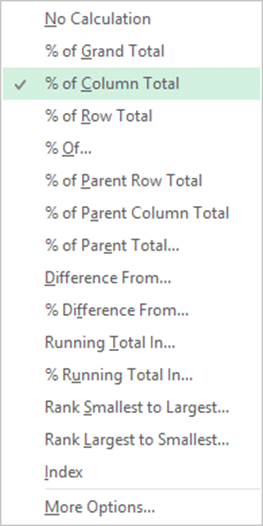The default action is No Calculation. That is actually wrong – technically speaking. There is SUM calculation already done. But in the context of showing values with respect to each other – no calculation is done. That is what it means.

Anyway. There is a very long list of options below it. Few options have been added in 2007, 2010 and 2013. So just go to that list and have a good hard look at each option. Read it and try to think what it must be doing.

Summarize by talks about individual value. Show Values As refers to all values.

## Showing percentage of total

There are three ways of doing it based upon what is 100%. % of grand total, column total or row total.

Consider this simple data – Segment and total amount by segment. This is without any additional calculation.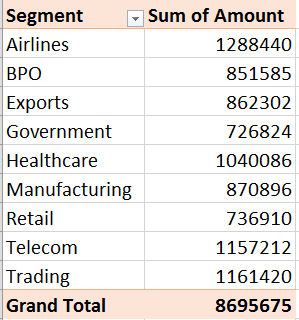In this case there is only one column. Therefore, % of column total and % of grand total will both give the same result. We will choose % of column total for now.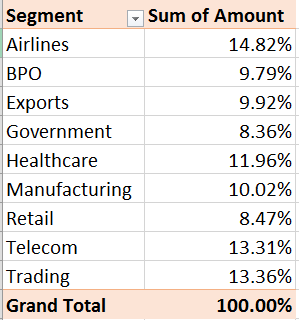So far so good. But unfortunately our original calculation is now REPLACED with the percentages. You wan to see the original as well.

No problem, drag and drop the Amount column again to the data area. You will get both.

Now let us add the Customer Type column in Column area – notice that the % of column total still continues to apply for each column.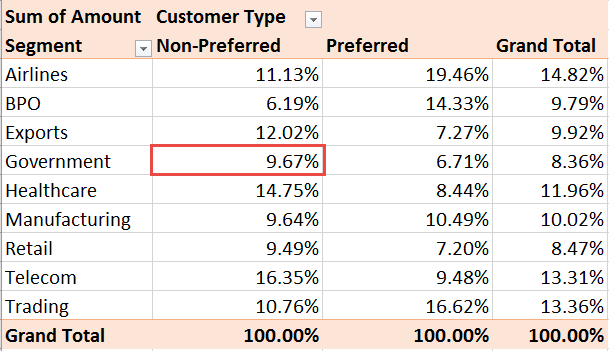If you had added formulas manually- you would have to manage this yourself! This is how we increase our work and don’t let Excel help you simplify your life.

## Interpreting the information

It is all nice – but how do you interpret it?

Focus on the data  which shows shows 9.67% for Government and Non-Preferred customers. What does it mean?

Of the total business done with non-preferred customers, the government customers contribute 9.67%.

Now let us change to Show Values As  – % of Row Total and see what changes. All the rows now show 100%. The value in that cell has now changed to 64.48%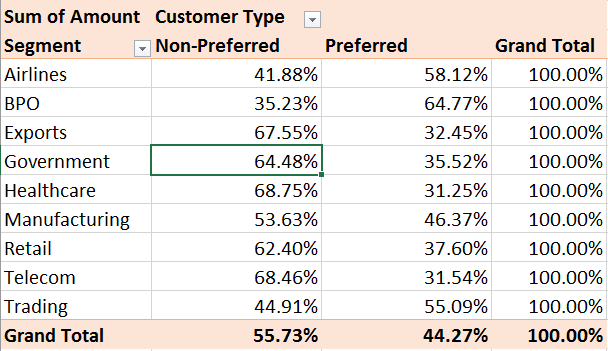Why did the value change? Our data has not changed at all. This is because now it is showing the ROW level total breakup. How do you interpret this?

Of all the business done with Government, 64.48% comes from Non-Preferred customers.

Now let us move to % of grand total. Notice that the only the grand total shows 100% now. The number changed to 5.39%.Of all the business we are doing – the contribution of Government business from Non-Preferred customers is 5.39%

## What did we learn?

Notice that the data has not changed. But each option gave us a different picture of the same data. This is called understanding!

This process is called converting data to useful information.

That is the crux of everything related to data analysis. We will explore more options from Show Values As in the next article.

***